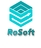### Count similar records in same table

Newbie question... I'm trying to write a formula to count the number of records in the same table which match a certain condition.  For example, in a table called "Customers", I want to create a calculation field that counts how many other customers have the same name as the current record.  I'm completely lost!  I've tried somethign like this, but with no luck:

`cnt('Customers'.'First Name' where 'First Name' = this.'First Name')`

I know that's horribly wrong, but it's where I'm starting, and I can't find any other info.

Moving on from there, I then need to add additional conditions (e.g. count people with same first name, who joined before me).  So then I can do things like add conditional running totals, etc.Follow this: first make a variable with the content of the field First Name in your form.

let c := 'First Name'

then we count all the records in the Customer table with the same first name and make it also a variable like this:

d:= cnt(select Customer where 'First Name' = c)

and now display the result like this:

d

So put this all together in a formula field:

`let c := 'First Name';`

`d:= cnt(select Customers where 'First Name'=c);`

`d`

Now you can do other stuff with the variabe d like e.g.

if d > 1 then

alert("There are " +d+" First Names the same")

end

Steven.

Thanks Steven!  Very helpful.  :-)

Dear all, I still do not get how to count numbers of table entries that are identical, based on a condition such as: IF ENTRY=Jim then count the entry

Thanks in advance

David

Hi David -

Can you give us a sample of your code you are trying to use?

Reply